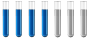## calculation of neutralization heat

Chemistry and homework help forum.

Organic Chemistry, Analytical Chemistry, Biochemistry, Physical Chemistry, Computational Chemistry, Theoretical Chemistry, High School Chemistry, Colledge Chemistry and University Chemistry Forum.

Share your chemistry ideas, discuss chemical problems, ask for help with scientific chemistry questions, inspire others by your chemistry vision!

Please feel free to start a scientific chemistry discussion here!

Discuss chemistry homework problems with experts!

Ask for help with chemical questions and help others with your chemistry knowledge!

Moderators: expert, ChenBeier, Xen

anto007
Sr. MemberPosts: 32
Joined: Tue Aug 10, 2021 10:13 am
Location: Italy

### calculation of neutralization heat

Hello everyone, I have this exercise: "The neutralization heat of HCl by NaOH is ΔH°r = -56.2 kJ / mol. How much heat is released when 125 mL of 1,750 M HCl is mixed with 195 mL of 0.667 M NaOH?"
Would you help me figure out how to fix it?
First I determined n
n(NaOH)=M*V= 0.667 mol/L*0.195 L=0.13 mol
n(HCl)=M*V=1.750 mol/L*0.125 L=0.219 mol

but i don't know how to continue, thanks a lot for your help
The mind is like a parachute, it only works if it opens. -A. Einstein
ChenBeier
Distinguished MemberPosts: 916
Joined: Wed Sep 27, 2017 7:25 am
Location: Berlin, Germany

### Re: calculation of neutralization heat

The unit of heat released is kJ/ mol. So what is the maximum mol what react according NaOH + HCl => NaCl + H2O ?
anto007
Sr. MemberPosts: 32
Joined: Tue Aug 10, 2021 10:13 am
Location: Italy

### Re: calculation of neutralization heat

NaOH + HCl => NaCl + H2O

1mol + 1 mol => 1 mol + 1 mol

right?
The mind is like a parachute, it only works if it opens. -A. Einstein
ChenBeier
Distinguished MemberPosts: 916
Joined: Wed Sep 27, 2017 7:25 am
Location: Berlin, Germany

### Re: calculation of neutralization heat

You have to calculate how much mol you have with the datas given. Do you you have 1:1 or something else. But you have to get the ratio 1:1.
anto007
Sr. MemberPosts: 32
Joined: Tue Aug 10, 2021 10:13 am
Location: Italy

### Re: calculation of neutralization heat

ah ok, I understand what you were asking methe total moles I calculated, adding n(NaOH) and n(HCl), are 0.349 mol
but they are not in a 1: 1 ratio, with these data NaOh is the limiting reagent.
Q=-ΔH°r (heat released)
Q=-ΔH°r*n= -(-56.2 kJ/mol)*0.13 mol= 7.306 kJ
it's correct?
Last edited by anto007 on Sat Aug 28, 2021 5:15 am, edited 1 time in total.
The mind is like a parachute, it only works if it opens. -A. Einstein
ChenBeier
Distinguished MemberPosts: 916
Joined: Wed Sep 27, 2017 7:25 am
Location: Berlin, Germany

### Re: calculation of neutralization heat

Correct.
anto007
Sr. MemberPosts: 32
Joined: Tue Aug 10, 2021 10:13 am
Location: Italy

### Re: calculation of neutralization heat

Great, thanks so muchThe mind is like a parachute, it only works if it opens. -A. Einstein